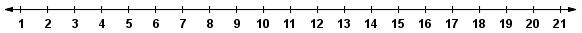Create a new printable
Answer key also includes questions
Answer key only gives the answers
No answer keyMath Worksheets

Sample - Click above to make a new math worksheet (PDF).
 Name _____________________________Date ___________________
Regroup Addition
Do you need to regroup? Write the difference.1.
Subtract:
15  -  6
Do you need to regroup?
 Yes No
What is the difference?

______
2.
Subtract:
15  -  1
Do you need to regroup?
 Yes No
What is the difference?

______
3.
Subtract:
14  -  2
Do you need to regroup?
 Yes No
What is the difference?

______
4.
Subtract:
12  -  9
Do you need to regroup?
 Yes No
What is the difference?

______
 5 * This is a pre-made sheet.Use the link at the top of the page for a printable page.
6.
Subtract:
11  -  0
Do you need to regroup?
 Yes No
What is the difference?

______
7.
Subtract:
19  -  1
Do you need to regroup?
 Yes No
What is the difference?

______
8.
Subtract:
13  -  8
Do you need to regroup?
 Yes No
What is the difference?

______
9.
Subtract:
18  -  9
Do you need to regroup?
 Yes No
What is the difference?

______
10.
Subtract:
16  -  8
Do you need to regroup?
 Yes No
What is the difference?

______

 Answer Key

Sample
This is only a sample worksheet.

Create a new printable
Answer key also includes questions
Answer key only gives the answers
No answer keyMath Worksheets